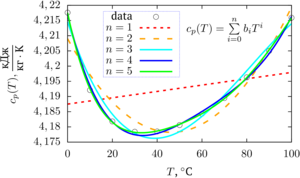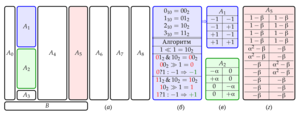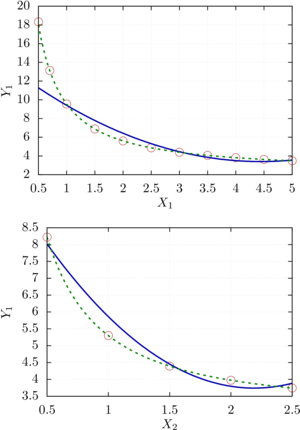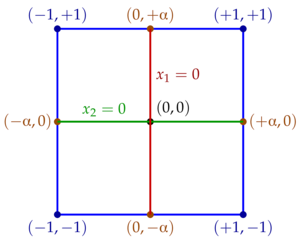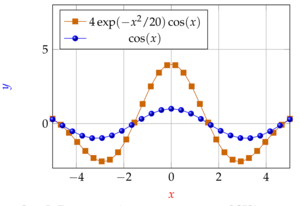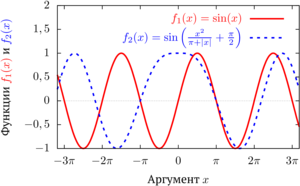ISSN 2658–5782
DOI 10.21662
Electronic Scientific Journalим. Р.Р. Мавлютова
УФИЦ РАНNasibullayev I.Sh. Application of free software for processing and visualization of scientific research results. Multiphase Systems. 16 (2021) 2. 58–71 (in Russian).
2021. Vol. 16. Issue 2, Pp. 58–71
URL: http://mfs.uimech.org/mfs2021.2.009,en
DOI: 10.21662/mfs2021.2.009
Application of free software for processing and visualization of scientific research results
Nasibullayev I.Sh.
Mavlyutov Institute of Mechanics UFRC RAS, Ufa, Russia

### Abstract

The paper presents an overview and examples of the use of free software for processing, analyzing and visualizing the results of computational and natural experiments. The implementation of two standard methods of approximation and data analysis is considered: the method of least squares and orthogonal central composite design. The first method allows the approximation of tabular data in the form of a polynomial of arbitrary degree. With an increase in the degree of the polynomial, the accuracy of the approximation increases, but, due to the growth of the condition number of the matrix, the correctness of the approximation decreases. It is shown that the normalization of the initial data makes it possible to increase the maximum order of the polynomial, in which there will be no loss of accuracy due to an ill-conditioned matrix. The implementation of the method in the Scilab programming language is proposed. The second method makes it possible to obtain a second-order approximation for an arbitrary number of variables, to estimate the linear and quadratic contributions of individual variables and their coupled interactions. A description of the method is presented and a scheme for constructing an extended experiment design matrix is proposed. The method is implemented in the C++ programming language, and a script written in the Scilab language is proposed to determine the error of the obtained approximation. To obtain a more accurate approximation, one can choose a function that takes into account the asymptotic features of the results of the computational experiment. An example of such an approximation is implemented in the computer algebra software wxMaxima. The presented software implementations of approximation methods additionally generate code in the Gnuplot and Scilab format, which increases efficiency for subsequent visualization or analysis of approximations. Examples of research visualization in the PGFPlots LATEX package and in the Gnuplot program using the epslatex terminal are given. Both approaches are convenient when presenting research results in the LATEX desktop publishing system.

Keywords

free software,
the method of least squares,
orthogonal central composite design,
Scilab,
wxMaxima,
Latex,
Gnuplot

## Article outline

The paper presents an overview and examples of the use of free software for processing, analyzing and visualizing the results of computational and natural experiments. The implementation of two standard methods of approximation and data analysis is considered: the method of least squares and orthogonal central composite design.

The first method allows the approximation of tabular data in the form of a polynomial of arbitrary degree. With an increase in the degree of the polynomial, the accuracy of the approximation increases, but, due to the growth of the condition number of the matrix, the correctness of the approximation decreases. It is shown that the normalization of the initial data makes it possible to increase the maximum order of the polynomial, in which there will be no loss of accuracy due to an ill-conditioned matrix. The implementation of the method in the Scilab programming language with source code generation for subsequent visualization of the approximation results using the Gnuplot sofrware is proposed.

The second method makes it possible to obtain a second-order approximation for an arbitrary number of variables, to estimate the linear and quadratic contributions of individual variables and their coupled interactions. A description of the method is presented and a scheme for constructing an extended experiment design matrix is proposed. For the convenience of processing the results of the experiment, the following structure of the extended matrix was chosen: columns with a dummy parameter, a full factorial experiment, star points and a central point; coded values for coupled interactions; quadratic terms; natural values of factors and initial data (parameters and results of the experiment); the error of the objective function at the points of the design; a row containing the coefficients of the polynomial of the orthogonal central composite design. The method is implemented in the C++ programming language with the generation of the resulting polynomial in the Scilab and Gnuplot formats. To determine the error of the objective function of the obtained approximation with respect to the initial data, a script written in the Scilab language is proposed. In a rare case (when the mathematical model of the object under study completely coincides with the polynomial used), the approximation does not allow extrapolation beyond the specified range - the farther the point is outside the data range, the higher the error and the approximation becomes inadequate to the experimental or simulation data. In order to obtain a sufficiently accurate and adequate approximation, it is recommended to divide a wide range of factor values into several parts and carry out orthogonal central composite design for each of them.

To obtain a more accurate approximation, one can choose a function that takes into account the asymptotic features of the results of the computational experiment. An example of such an approximation is implemented in the computer algebra software wxMaxima. The presented software implementations of approximation methods additionally generate code in the Gnuplot and Scilab format, which increases efficiency for subsequent visualization or analysis of approximations.

Examples of research visualization in the PGFPlots LaTeX package and in the Gnuplot program using the epslatex terminal are given. Both approaches are convenient when presenting research results in the LaTeX desktop publishing system.

## References

1. MATLAB, MathWorks, MATLAB & Simulink.
https://www.mathworks.com/products/matlab.html (accessed: 07.12.2021)
2. Scilab.
https://www.scilab.org/ (accessed: 07.12.2021)
3. Campbell S.L., Chancelier J.-Ph., Nikoukhah R. Modeling and Simulation in Scilab/Scicos with ScicosLab 4.4. 2010. Springer, New York, NY. 330 p.
DOI: 10.1007/978-1-4419-5527-2
4. GNU Octave.
http://www.gnu.org/software/octave/ (accessed: 07.12.2021)
5. Alekseyev Ye.R., Chesnokova O.V. [Introduction to Octave for engineers and mathematicians] Vvedeniye v Octave dlya inzhenerov i matematikov. M.: ALT Linux, 2012. 368 p. (in Russian).
http://www.altlinux.org/images/0/07/OctaveBook.pdf (accessed: 07.12.2021)
6. Maxima, a computer algebra system.
https://maxima.sourceforge.io/ (accessed: 07.12.2021)
7. Stakhin N.A. [Fundamentals of working with the system of analytical (symbolic) calculations Maxima. Tutorial] Osnovy raboty s sistemoy analiticheskikh (simvolnykh) vychisleniy Maxima. Uchebnoye posobiye. M.: 2008. 86 p. (in Russian).
http://www.uneex.ru/static/MethodBooks_Maxima/Maxima.pdf (accessed: 07.12.2021)
8. Wolfram Mathematica: Modern Technical Computing.
http://www.wolfram.com/mathematica (accessed: 07.12.2021)
9. Maplesoft: software for mathematics, online learning, engineering.
http://www.maplesoft.com/ (accessed: 07.12.2021)
10. wxMaxima.
https://wxmaxima-developers.github.io/wxmaxima/ (accessed: 07.12.2021)
11. Nasibullayev I.Sh. [Application of free software FreeFem++/Gmsh and FreeCAD/CalculiX for simulation of static elasticity problems] Primeneniye svobodnykh programm FreeFem++/Gmsh i FreeCAD/CalculiX dlya modelirovaniya staticheskikh strukturnykh zadach // Mnogofaznyye sistemy [Multiphase Systems]. 2020. Vol. 15, N 3–4. Pp. 183–200. (in Russian).
DOI: 10.21662/mfs2020.3.129
12. Bretscher O. Linear Algebra With Applications (3rd ed.). 1995. Upper Saddle River, NJ: Prentice Hall.
13. Durakovic B. Design of Experiments Application, Concepts, Examples: State of the Art. // Periodicals of Engineering and Natural Scinces. 2017. Vol. 5, No. 3. Pp. 421–439.
DOI: 10.21533/pen.v5i3.145
14. Adler Yu.P., Markova Ye.V., Granovskiy Yu.V. [Design of experiment in the search for optimal conditions] Planirovaniye eksperimenta pri poiske optimalnykh usloviy. M.: Nauka, 1976. 280 p. (in Russian).
15. LaTeX — a document preparation system.
http://www.latex-project.org/ (accessed: 07.12.2021)
16. Kotelnikov I.A., Chebotayev P.Z. [LaTeX in Russian] LaTeX po-russki. SPb.: “Korona-Vek”. 2011. 496 p. (in Russian).
17. van Dongen M.R.C. LaTeX and Friends. 2012. Springer-Verlag. Berlin, Heidelberg. 300 p.
DOI: 10.1007/978-3-642-23816-1
18. Microsoft.
https://www.microsoft.com/ (accessed: 07.12.2021)
19. Feuersänger C. Manual for Package PGFPlots, 2D/3D Plots in LATEX, Version 1.18.
http://pgfplots.sourceforge.net/pgfplots.pdf (accessed: 07.12.2021)
20. The TikZ and PGF Packages. Manual for version 3.1.9a. Ed. by Till Tantau. Universitä zu Lübeck, 2021.
https://mirror.truenetwork.ru/CTAN/graphics/pgf/base/doc/pgfmanual.pdf (accessed: 07.12.2021)
21. PSTricks.
https://tug.org/PSTricks/main.cgi (accessed: 07.12.2021)
22. Gnuplot.
http://www.gnuplot.info/ (accessed: 07.12.2021)
23. Origin — data analysis and graphing software.
http://www.originlab.com/ (accessed: 07.12.2021)
24. CTAN: package listings.
https://ctan.org/pkg/listings (accessed: 07.12.2021)
25. CTAN: package beamer.
https://ctan.org/pkg/beamer (accessed: 07.12.2021)
26. Nasibullayev I.Sh. [Analytical analysis of operating mode switching in a 2D model of a fluid cooling system of the micro-gripper] Analiticheskiy analiz pereklyucheniya rabochego rezhima v dvumernoy modeli sistemy zhidkostnogo okhlazhdeniya mikrozakhvata // Vestnik UGATU. 2021. Vol. 25, N. 3 (93). Pp. 120–131. (in Russian).
DOI: 10.54708/19926502_2021_25393120
27. Volkov A.I., Zharskiy I.M. [Big chemical reference book] Bolshoy khimicheskiy spravochnik. M.: Sovremennaya shkola [Modern school]. 2005. 608 p. (in Russian).
28. Khuri A.I., Cornell J.A. Response surface, design and analysis, 2nd edition, Marcel Dekker Inc., New York. 1996.
29. Nasibullayev I.Sh. [The development of a computer model for the main element of the fuel metering unit] Razrabotka kompyuternoy modeli osnovnogo elementa agregata dozirovaniya topliva. Vychislitelnye tehnologii [Computational Technologies]. 2016. V. 21, No. 2. Pp. 26–41. (In Russian).
http://www.ict.nsc.ru/jct/getfile.php?id=1724
30. Oyejola B.A., Nwanya J.C. Selecting the Right Central Composite Design. International Journal of Statistics and Applications. 2015. Vol. 5, No. 1. Pp. 21–30.
DOI: 10.5923/j.statistics.20150501.04
31. Nasibullayev I.Sh., Darintsev O.V. [Computer 2D modelling of a micro-grip fluid cooling system] Komp’yuternoye dvumernoye mod elirovaniye sistemy zhidkostnogo okhlazhdeniya mikrozakhvata // Vychislitelnye tehnologii [Computational Technologies]. 2021. Vol. 26. N. 2. Pp. 4–20. (In Russian).
DOI: 10.25743/ICT.2021.26.2.002
32. Shklyar V.N. [Design of experiment and results processing] Planirovaniye eksperimenta i obrabotka rezultatov. Izdatelstvo Tomskogo politekhnicheskogo universiteta [Tomsk Polytechnic University Publishing House]. 2010. (In Russian).
33. Ghostscript.
https://www.ghostscript.com/ (accessed: 07.12.2021)